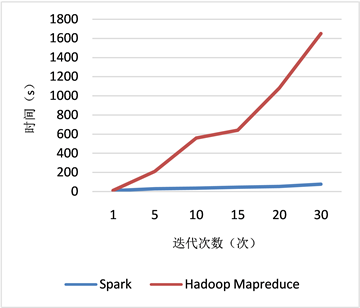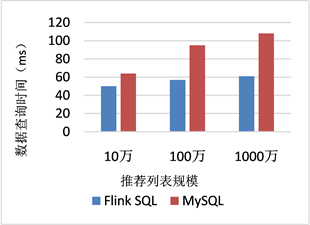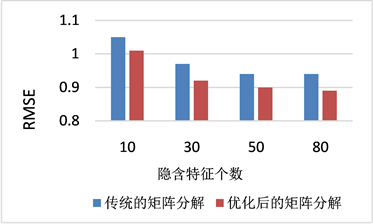Abstract: Although progress and rapid development of the Internet also brought a lot of network data flow, the following is the comprehensive storage of data, data comprehensive calculation and data analysis and many other problems. With the complexity and diversification of various business systems, the requirements for the effectiveness of data analysis have become increasingly high. In the past, most offline analysis commonly used is no longer applicable to today’s production needs. Now the data recommendation system is requested to have a higher demand in real time. As a popular recommendation algorithm at present, the recommendation algorithm based on matrix decomposition is obviously superior to other algorithms in terms of accuracy and accuracy of prediction. However, the traditional matrix decomposition method has the problems of slow computation speed and insufficient computation resources when dealing with large-scale data. As a popular streaming data processing framework, Flink big data framework has obvious advantages in iterative computation and streaming data processing. In this paper, matrix decomposition method is combined with Flink processing. On the basis of the original matrix decomposition recommendation algorithm, an optimization model of matrix decomposition algorithm based on Flink is proposed to solve the bottleneck of matrix decomposition in the big data environment.

1. 引言

“大数据”技术近几年来快速发展，它不仅是一个企业发展趋势，也是一个改变了人类日常生活的重大技术创新。大数据对整个行业以及用户的整合重要性也日益突出，进行高度智能化商业决策，已成为企业脱颖而出的重要关键，越来越多的中国企业已经开始针对企业大数据技术进行企业战略整合布局，重新正确定义自己的企业核心战略竞争力。

3. 矩阵分解

${R}^{\prime }={U}^{\text{T}}\cdot S\cdot V$ (1)

${{R}^{\prime }}_{f}\left(u,i\right)={U}_{f}^{\text{T}}\cdot {S}_{f}\cdot {V}_{f}$ (2)

4. 问题分析

5. 算法优化

5.1. 具体步骤

5.1.1. 相似度计算

$sim\left(i,j\right)=\frac{{\sum }_{c\in {I}_{ij}}\left({R}_{i,C}-{R}_{i}\right)\left({R}_{j,C}-{R}_{j}\right)}{\sqrt{{\sum }_{c\in {I}_{i}}{\left({R}_{i,C}-{R}_{i}\right)}^{2}}\sqrt{{\sum }_{c\in {I}_{j}}{\left({R}_{j,C}-{R}_{j}\right)}^{2}}}$ (3)

5.1.2. 设计目标函数

$\begin{array}{c}f\left(U,M\right)=\underset{\left(i,j\right)\in I}{\sum }{\left({r}_{ij}-{u}_{i}{m}_{j}^{\text{T}}\right)}^{2}+\underset{i\in {I}_{j}}{\sum }{\left({u}_{ki}-\frac{{\sum }_{{u}_{p}\in KNN\left({u}_{i}\right)}si{m}_{{u}_{i}{u}_{p}}{u}_{kp}}{{\sum }_{{u}_{p}\in KNN\left({u}_{i}\right)}si{m}_{{u}_{i}{u}_{p}}}\right)}^{2}\\ \text{\hspace{0.17em}}\text{ }\text{ }+\underset{j\in {I}_{i}}{\sum }{\left({m}_{kj}-\frac{{\sum }_{{m}_{q}\in KNN\left({m}_{j}\right)}si{m}_{{m}_{i}{m}_{p}}{m}_{kp}}{{\sum }_{{m}_{q}\in KNN\left({m}_{j}\right)}si{m}_{{m}_{i}{m}_{p}}}\right)}^{2}+\lambda \left({p}_{u}^{2}+{q}_{i}^{2}\right)\end{array}$ (4)

5.1.3. 求解目标函数

${}_{2}{}^{1}{}_{\partial {u}_{ki}}{}^{\partial f}=0,\forall i,k⇒$ $\underset{j\in {I}_{i}}{\sum }{\left({u}_{i}^{\text{T}}{m}_{j}-{r}_{ij}\right)}^{2}+\left({u}_{ki}-\frac{{\sum }_{{u}_{p}\in KNN\left({u}_{i}\right)}si{m}_{{u}_{i}{u}_{p}}{u}_{kp}}{{\sum }_{{u}_{p}\in KNN\left({u}_{i}\right)}si{m}_{{u}_{i}{u}_{p}}}\right)+\lambda {n}_{ui}{n}_{ki}=0,\forall i,k$(5)

$⇒{u}_{i}={\left({M}_{{I}_{I}}{M}_{{I}_{I}}^{\text{T}}+\left(\lambda {n}_{ui}+1\right)E\right)}^{-1}×\left({M}_{{I}_{i}}{R}^{\text{T}}\left(i,{I}_{i}\right)+\frac{{\sum }_{{u}_{p}\in KNN\left({u}_{i}\right)}si{m}_{{u}_{i}{u}_{p}}{u}_{kp}}{{\sum }_{{u}_{p}\in KNN\left({u}_{i}\right)}si{m}_{{u}_{i}{u}_{p}}}\right),\forall i$ (6)

${m}_{j}={\left({M}_{{I}_{I}}{M}_{{I}_{I}}^{\text{T}}+\left(\lambda {n}_{ui}+1\right)E\right)}^{-1}×\left({M}_{{I}_{i}}{R}^{\text{T}}\left(j,{I}_{j}\right)+\frac{{\sum }_{{m}_{q}\in KNN\left({m}_{j}\right)}si{m}_{{m}_{j}{m}_{q}}{m}_{kp}}{{\sum }_{{m}_{q}\in KNN\left({m}_{j}\right)}si{m}_{{m}_{j}{m}_{q}}}\right),\forall i$ (7)

6. 实证分析

6.1. 数据来源及说明

6.2. 实验涉及

6.3. 测评结果Figure 1. Comparison of model training timeFigure 2. Comparison of training time of matrix decomposition model in different iteration timesFigure 3. Comparison of Flink SQL and MySQL data query timeFigure 4. RMSE values of different implicit eigenvalues

7. 结论

 张延彬. 基于移动通信行业的大数据服务研究[J]. 电信工程技术与标准化, 2016, 29(2): 44-47.

 古来, 黄俊, 张若凡, 等. 结合多信息的概率矩阵分解模型[J]. 软件导刊, 2018, 17(9): 67-71.

 翁小兰, 王志坚. 协同过滤推荐算法研究进展[J]. 计算机工程与应用, 2018, 54(1): 25-31.

 孟利民, 赵维, 应颂翔. 评分预测问题中个性化推荐模型的研究[J]. 浙江工业大学学报, 2016, 180(2): 119-123.

 王圣涛, 郝龙飞, 贾洁民. 一种基于NSGA-II的协同过滤推荐算法[J]. 电子产品世界, 2016(2): 57-60.

 冯洋. 基于改进的奇异值分解的红外弱小目标检测[J]. 激光技术, 2016, 40(3): 335-338.

 张宇, 程久军. 基于MapReduce的矩阵分解推荐算法研究[J]. 计算机科学, 2013(1): 19-21.

 王振军, 黄瑞章. 基于Spark的矩阵分解与最近邻融合的推荐算法[J]. 计算机系统应用, 2017, 26(4): 124-129.

 谢人强, 陈震. 基于共同评分项和权重计算的推荐算法研究[J]. 计算机技术与发展, 2016, 26(9): 69-72.

 李昆仑, 郭昌隆, 关立伟. 一种融合近邻用户影响力的矩阵分解推荐算法[J]. 小型微型计算机系统, 2018, 39(1): 37-41.

 任彩霞. 一种改进的缓解推荐系统物品冷启动的方法[J]. 软件, 2016(8): 11-15.

 Yazidi, A.E., Azizi, M.S., Benlachmi, Y., et al. (2021) Apache Hadoop-MapReduce on YARN Framework Latency. Procedia Computer Science, 184, 803-808.
https://doi.org/10.1016/j.procs.2021.03.100

 包维宁, 任钦正, 李瑞明, 等. 一种基于Flink的日志流式处理方法及系统[P]. CN111177193A. 2020.

 杰诚, 郑少明, 郑乐乐, 等. 一种基于Flink SQL的数据处理方法, 装置, 存储介质[P]. CN111026779A. 2020.

Top